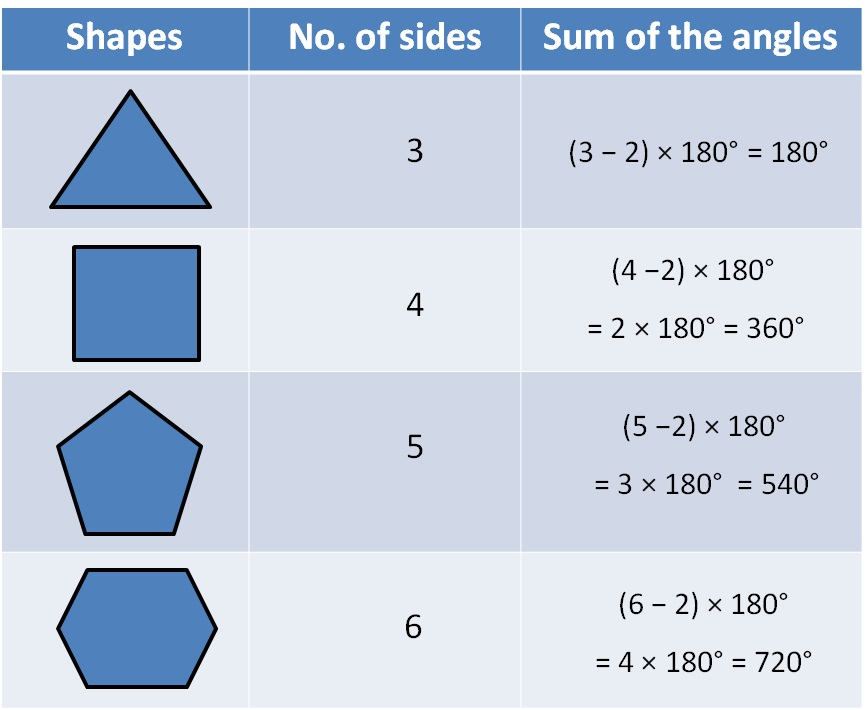Sum of angles of a polygon = (n – 2) × 180°

Let’s look at some examples1. Chapter 3 Class 8 Understanding Quadrilaterals
2. Concept wise
3. Polygons